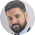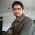# Difference between Permutations and Combinations

Often students confuse between Permutations and Combinations. While both are used to find ways to do a specific job, in permutation order is important whereas in combinations, order is not important

### Lets take a simple example

Question - How many 4 color stripes flags can be made using six different colors. Repetition is not allowed.

There is a proper order and there are restrictions so we will use permutation.

nPr = n!/(n-r)! = 6!/(6-4)! = 6!/2! = 6*5*4**3 = 360

Question - How many 4 color stripes flags can be made using six different colors. Repetition is allowed.

As repetition is allowed and there is no proper and restrictions, we can use combinations here
nCr = n!/r(n-r)! = 6!/

If the order doesn't matter, it is a Combination. If the order does matter it is a Permutation.

You can practice this chapter on Testpanda.com#### What's trending in BankExamsToday

Smart Prep Kit for Banking Exams by Ramandeep Singh - Download here1.why are u not making videos dese days........bro??

1.I am making, these are part of DVD pack. Will upload some of them soon.

2.please give all sort of topic related to permutation combination probability and geometry

3.Sir, kindly post some 'General English' notes with proper explanation for each topics.Thanks

4.let take example of three books A,B and C... if we have to choose two books at a time ....then possible cases are....AB,AC,BC,BA,CB and CA .....if it is permutation(i.e. arrangement) thn total no. of permutation are 6.....but if only we have to select i.e only combine two books we use combination...and no. of combination are 3 .that are AB,BC and AC...in combination order doesn't matter....

Thanks for commenting. Follow us on Telegram. Search BankExamsToday